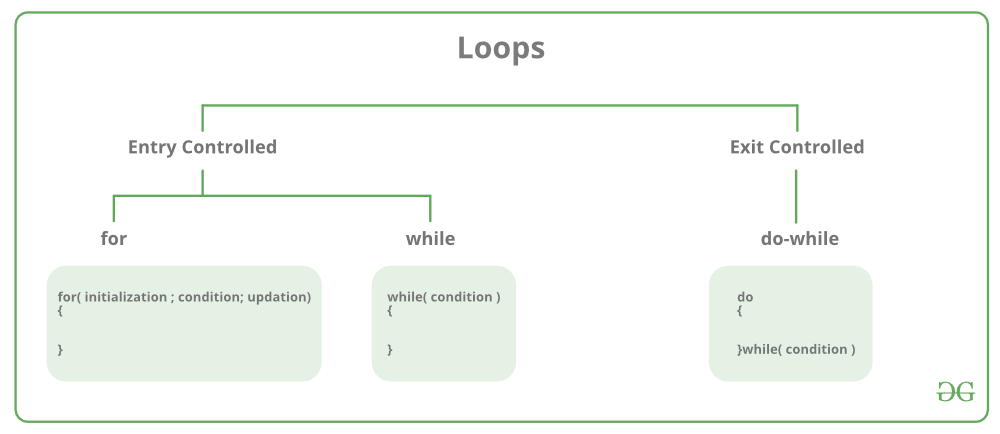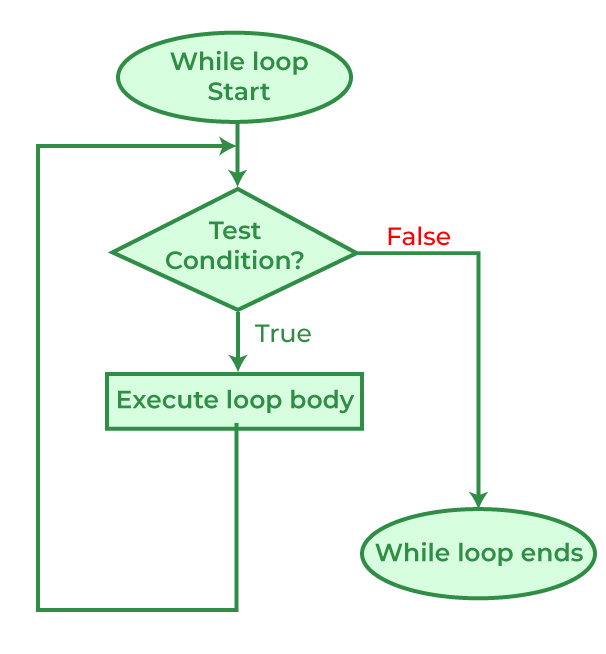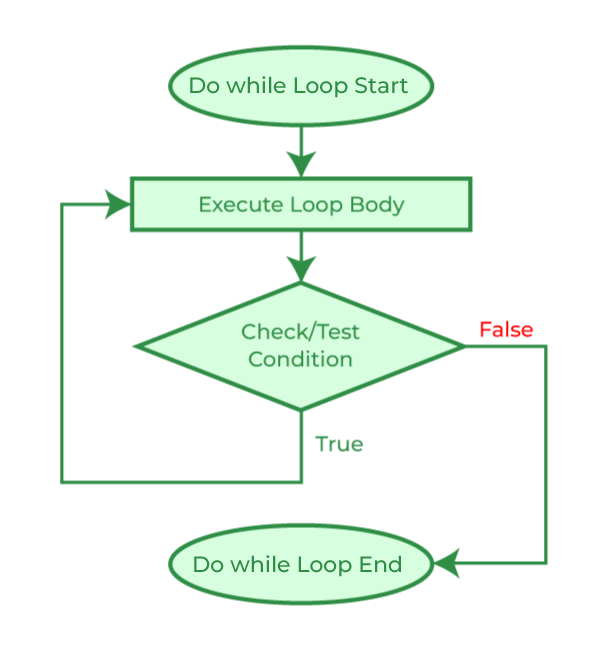# C – Loops

Loops in programming are used to repeat a block of code until the specified condition is met. A loop statement allows programmers to execute a statement or group of statements multiple times without repetition of code.

## C

 `// C program to illustrate need of loops` `#include ` ` `  `int` `main()` `{` `    ``printf``( ``"Hello World\n"``);` `    ``printf``( ``"Hello World\n"``);` `    ``printf``( ``"Hello World\n"``);` `    ``printf``( ``"Hello World\n"``);` `    ``printf``( ``"Hello World\n"``);` `    ``printf``( ``"Hello World\n"``);` `    ``printf``( ``"Hello World\n"``);` `    ``printf``( ``"Hello World\n"``);` `    ``printf``( ``"Hello World\n"``);` `    ``printf``( ``"Hello World\n"``);` `     `  `    ``return` `0;` `}`

Output

```Hello World
Hello World
Hello World
Hello World
Hello World
Hello World
Hello World
Hello World
Hello World
Hello World```

There are mainly two types of loops in C Programming:

1. Entry Controlled loops: In Entry controlled loops the test condition is checked before entering the main body of the loop. For Loop and While Loop is Entry-controlled loops.
2. Exit Controlled loops: In Exit controlled loops the test condition is evaluated at the end of the loop body. The loop body will execute at least once, irrespective of whether the condition is true or false. do-while Loop is Exit Controlled loop.## for Loop

for loop in C programming is a  repetition control structure that allows programmers to write a loop that will be executed a specific number of times. for loop enables programmers to perform n number of steps together in a single line.

Syntax:

```for (initialize expression; test expression; update expression)
{
//
// body of for loop
//
}```

Example:

```for(int i = 0; i < n; ++i)
{
printf("Body of for loop which will execute till n");
}```

In for loop, a loop variable is used to control the loop. Firstly we initialize the loop variable with some value, then check its test condition. If the statement is true then control will move to the body and the body of for loop will be executed. Steps will be repeated till the exit condition becomes true. If the test condition will be false then it will stop.

• Initialization Expression: In this expression, we assign a loop variable or loop counter to some value. for example: int i=1;
• Test Expression: In this expression, test conditions are performed. If the condition evaluates to true then the loop body will be executed and then an update of the loop variable is done. If the test expression becomes false then the control will exit from the loop. for example, i<=9;
• Update Expression: After execution of the loop body loop variable is updated by some value it could be incremented, decremented, multiplied, or divided by any value.

for loop Equivalent Flow Diagram:Example:

## C

 `// C program to illustrate for loop` `#include ` ` `  `// Driver code` `int` `main()` `{` `  ``int` `i = 0;` `  `  `  ``for` `(i = 1; i <= 10; i++)` `  ``{` `    ``printf``( ``"Hello World\n"``);   ` `  ``}` `  ``return` `0;` `}`

Output

```Hello World
Hello World
Hello World
Hello World
Hello World
Hello World
Hello World
Hello World
Hello World
Hello World```

## While Loop

While loop does not depend upon the number of iterations. In for loop the number of iterations was previously known to us but in the While loop, the execution is terminated on the basis of the test condition. If the test condition will become false then it will break from the while loop else body will be executed.

Syntax:

```initialization_expression;

while (test_expression)
{
// body of the while loop

update_expression;
}```

Flow Diagram for while loop:## C

 `// C program to illustrate ` `// while loop` `#include ` ` `  `// Driver code` `int` `main()` `{` `  ``// Initialization expression` `  ``int` `i = 2; ` ` `  `  ``// Test expression` `  ``while``(i < 10)` `  ``{` `    ``// loop body` `    ``printf``( ``"Hello World\n"``);   ` `    `  `    ``// update expression` `    ``i++;` `  ``} ` `  `  `  ``return` `0;` `}`

Output

```Hello World
Hello World
Hello World
Hello World
Hello World
Hello World
Hello World
Hello World```

## do-while Loop

The do-while loop is similar to a while loop but the only difference lies in the do-while loop test condition which is tested at the end of the body. In the do-while loop, the loop body will execute at least once irrespective of the test condition.

Syntax:

```initialization_expression;
do
{
// body of do-while loop

update_expression;

} while (test_expression);```## C

 `// C program to illustrate ` `// do-while loop` `#include ` ` `  `// Driver code` `int` `main()` `{` `  ``// Initialization expression` `  ``int` `i = 2; ` `  `  `  ``do` `  ``{` `    ``// loop body` `    ``printf``( ``"Hello World\n"``);   ` ` `  `    ``// Update expression` `    ``i++;` `    `  `    ``// Test expression` `  ``}  ``while` `(i < 1);   ` `  `  `  ``return` `0;` `}`

Output

`Hello World`

Above program will evaluate (i<1) as false since i = 2. But still, as it is a do-while loop the body will be executed once.

## Loop Control Statements

Loop control statements in C programming are used to change execution from its normal sequence.

## Infinite Loop

An infinite loop is executed when the test expression never becomes false and the body of the loop is executed repeatedly. A program is stuck in an Infinite loop when the condition is always true. Mostly this is an error that can be resolved by using Loop Control statements.

Using for loop:

## C

 `// C program to demonstrate infinite` `// loops using for loop` `#include ` ` `  `// Driver code` `int` `main ()` `{` `  ``int` `i;` `  `  `  ``// This is an infinite for loop ` `  ``// as the condition expression ` `  ``// is blank` `  ``for` `( ; ; )` `  ``{` `    ``printf``(``"This loop will run forever.\n"``);` `  ``}` `  `  `  ``return` `0;` `}`

Output

```This loop will run forever.
This loop will run forever.
This loop will run forever.
...```

Using While loop:

## C

 `// C program to demonstrate ` `// infinite loop using while ` `// loop` `#include ` ` `  `// Driver code` `int` `main() ` `{` `  ``while` `(1)` `    ``printf``(``"This loop will run forever.\n"``);` `  ``return` `0;` `}`

Output

```This loop will run forever.
This loop will run forever.
This loop will run forever.
...```

Using the do-while loop:

## C

 `// C program to demonstrate ` `// infinite loop using do-while ` `// loop` `#include `   `// Driver code` `int` `main()` `{` `  ``do` `  ``{` `    ``printf``(``"This loop will run forever.\n"``);` `  ``} ``while` `(1);` `  `  `  ``return` `0;` `}`

Output

```This loop will run forever.
This loop will run forever.
This loop will run forever.
...```

Whether you're preparing for your first job interview or aiming to upskill in this ever-evolving tech landscape, GeeksforGeeks Courses are your key to success. We provide top-quality content at affordable prices, all geared towards accelerating your growth in a time-bound manner. Join the millions we've already empowered, and we're here to do the same for you. Don't miss out - check it out now!

Previous
Next